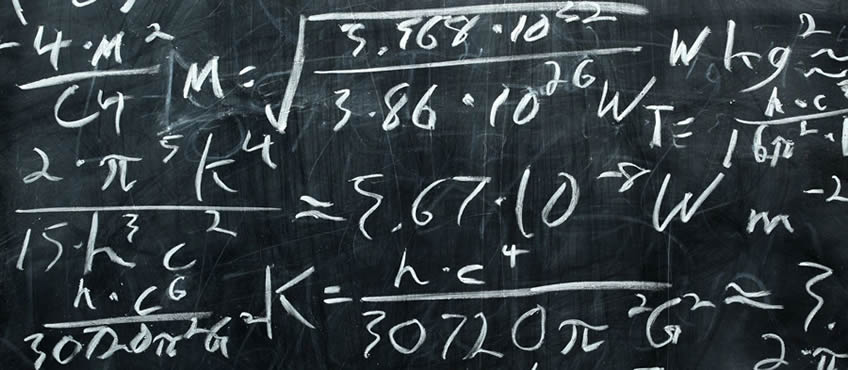Education

# Working with PercentagesA percentage is a measurement of an amount out of every hundred. We recommend using percentages to formulate your products. This is because it is not dependent on the quantity you are making it is consistent.

Working with percentages is an essential part of formulating your product. When manufacturing your product the quantity you produce will vary over time. For example, if you find that one of your products sells more than the others you may have to produce bigger batches. In this case you will need to have your formula in percentages, so you can work out the quantity of each ingredient required for producing the same product.Percentages

What is a Percentage?

A percentage is a measurement of an amount out of every hundred. We recommend using percentages to formulate your products. This is because it is not dependent on the quantity you are making it is consistent.

The basic calculation for a percentage is:

Amount used divided by the total product amount times by 100.

So if you were making 500g of product and one ingredient was 100g you would calculate:

100 / 500 = 0.2

0.2 x 100 = 20

So 100 is 20% of 500

To work out how many grams you would need when you know the percentage you reverse the calculation. So:

20 / 100 = 0.2

0.2 x 500 = 100

Once you know the percentage it is easy to calculate how many grams you need, however much you are making. If you decided to make 820g of product instead of 500 you could apply the same calculation to work out what 20% is you would calculate:

20 / 100 = 0.2

0.2 x 820 = 164

So you would need 164g of that ingredient We have created a simple spreadsheet to help you to do your calculations of percentages. You just need to edit the boxes coloured in green and the spreadsheet will calculate the answers for you.